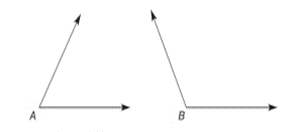Chapter 3.4, Problem 9E### Elementary Geometry for College St...

6th Edition
Daniel C. Alexander + 1 other
ISBN: 9781285195698

#### Solutions

Chapter
Section### Elementary Geometry for College St...

6th Edition
Daniel C. Alexander + 1 other
ISBN: 9781285195698
Textbook Problem
1 views

# In Exercises 7 to12, use the angle provided to perform the constructions.Construct an angle that has one half the measure of ∠ A .

To determine

To construct:

An angle that has one half of the measure of A.

Explanation

Construction:

Acute A is given.

Figure (1)

Step (1):

Consider the given acute A.Cut an arc from point A intersecting both rays at point B and C.

Figure (2)

Step (2):

Now, cut arcs of equal length from point B and C that intersects at point D.

### Still sussing out bartleby?

Check out a sample textbook solution.

See a sample solution

#### The Solution to Your Study Problems

Bartleby provides explanations to thousands of textbook problems written by our experts, many with advanced degrees!

Get Started

#### Find the median for the following set of scores: 1, 9, 3, 6, 4, 3, 11, 10

Essentials of Statistics for The Behavioral Sciences (MindTap Course List)

#### Simplify: 3656

Elementary Technical Mathematics

#### In Problems 33 and 34, find the particular solution to each differential equation.

Mathematical Applications for the Management, Life, and Social Sciences

#### The graph of is: a) b) c) d)

Study Guide for Stewart's Multivariable Calculus, 8th

#### Solving an Equation In Exercises11-26, solve for x. e2x=e5

Calculus: Early Transcendental Functions (MindTap Course List)